tensorflow 订阅
TensorFlow™是一个基于数据流编程（dataflow programming）的符号数学系统，被广泛应用于各类机器学习（machine learning）算法的编程实现，其前身是谷歌的神经网络算法库DistBelief   。Tensorflow拥有多层级结构，可部署于各类服务器、PC终端和网页并支持GPU和TPU高性能数值计算，被广泛应用于谷歌内部的产品开发和各领域的科学研究 [1-2]  。TensorFlow由谷歌人工智能团队谷歌大脑（Google Brain）开发和维护，拥有包括TensorFlow Hub、TensorFlow Lite、TensorFlow Research Cloud在内的多个项目以及各类应用程序接口（Application Programming Interface, API）   。自2015年11月9日起，TensorFlow依据阿帕奇授权协议（Apache 2.0 open source license）开放源代码   。 展开全文TensorFlow™是一个基于数据流编程（dataflow programming）的符号数学系统，被广泛应用于各类机器学习（machine learning）算法的编程实现，其前身是谷歌的神经网络算法库DistBelief   。Tensorflow拥有多层级结构，可部署于各类服务器、PC终端和网页并支持GPU和TPU高性能数值计算，被广泛应用于谷歌内部的产品开发和各领域的科学研究 [1-2]  。TensorFlow由谷歌人工智能团队谷歌大脑（Google Brain）开发和维护，拥有包括TensorFlow Hub、TensorFlow Lite、TensorFlow Research Cloud在内的多个项目以及各类应用程序接口（Application Programming Interface, API）   。自2015年11月9日起，TensorFlow依据阿帕奇授权协议（Apache 2.0 open source license）开放源代码   。

1.12.0/2018年10月9日

Linux，macOS，Windows

beta/2015年11月9日

iOS，Android，Web

TensorFlow

Python，C++，CUDA
TensorFlow背景

• ## 写给初学者的Tensorflow介绍

万次阅读 多人点赞 2018-04-16 17:07:14

注：这是一篇翻译的文章，原文地址：https://towardsdatascience.com/a-beginner-introduction-to-tensorflow-part-1-6d139e038278

本文的主要目的是为TensorFlow提供一个对初学者友好的介绍，我假设您已经知道一些python知识。 TensorFlow的核心组件是通过边遍历所有节点的计算图和张量。我们来逐个简单介绍一下。

张量(Tensor)在数学上，张量是N维向量，这意味着张量可以用来表示N维数据集。上面的图有点复杂，难以理解。我们看看它的简化版本：上图显示了一些简化的张量。随着维度的不断增加，数据表示将变得越来越复杂。例如，一个3x3的张量，我可以简单地称它为3行和列的矩阵。如果我选择另一个形式的张量（1000x3x3），我可以称之为一个向量或一组1000个3x3的矩阵。在这里我们将（1000x3x3）称为张量的形状或尺寸。张量可以是常数也可以是变量。

计算图(流, flow)

现在我们理解了Tensor的含义，是时候了解流(Flow)了。流是指一个计算图或简单的一个图，图不能形成环路，图中的每个节点代表一个操作，如加法、减法等。每个操作都会导致新的张量形成。上图展示了一个简单的计算图，所对应的表达式为：

e = (a+b)x(b+1)

计算图具有以下属性：

叶子顶点或起始顶点始终是张量。意即，操作永远不会发生在图的开头，由此我们可以推断图中的每个操作都应该接受一个张量并产生一个新的张量。同样，张量不能作为非叶子节点出现，这意味着它们应始终作为输入提供给操作/节点。
计算图总是以层次顺序表达复杂的操作。通过将a + b表示为c，将b + 1表示为d，可以分层次组织上述表达式。 因此，我们可以将e写为：

e = (c)x(d) 这里 c = a+b 且 d = b+1.

以反序遍历图形而形成子表达式，这些子表达式组合起来形成最终表达式。
当我们正向遍历时，遇到的顶点总是成为下一个顶点的依赖关系，例如没有a和b就无法获得c，同样的，如果不解决c和d则无法获得e。
同级节点的操作彼此独立，这是计算图的重要属性之一。当我们按照图中所示的方式构造一个图时，很自然的是，在同一级中的节点，例如c和d，彼此独立，这意味着没有必要在计算d之前计算c。 因此它们可以并行执行。

计算图的并行

上面提到的最后一个属性当然是最重要的属性之一。它清楚地表明，同级的节点是独立的，这意味着在c被计算之前不需空闲，可以在计算c的同时并行计算d。Tensorflow充分利用了这个属性。

分布执行

Tensorflow允许用户使用并行计算设备更快地执行操作。计算的节点或操作自动调度进行并行计算。这一切都发生在内部，例如在上图中，可以在CPU上调度操作c，在GPU上调度操作d。下图展示了两种分布式执行的过程：第一种是单个系统分布式执行，其中单个Tensorflow会话（将在稍后解释）创建单个worker，并且该worker负责在各设备上调度任务。在第二种系统下，有多个worker，他们可以在同一台机器上或不同的机器上，每个worker都在自己的上下文中运行。在上图中，worker进程1运行在独立的机器上，并调度所有可用设备进行计算。

计算子图

子图是主图的一部分，其本身就是计算图。例如，在上面的图中，我们可以获得许多子图，其中之一如下所示上面的图是主图的一部分，从属性2我们可以说子图总是表示一个子表达式，因为c是e的子表达式。 子图也满足最后一个属性。同一级别的子图也相互独立，可以并行执行。因此可以在一台设备上调度整个子图。上图解释了子图的并行执行。这里有2个矩阵乘法运算，因为它们都处于同一级别，彼此独立，这符合最后一个属性。由于独立性的缘故，节点安排在不同的设备gpu_0和gpu_1上。

在worker之间交换数据

现在我们知道Tensorflow将其所有操作分配到由worker管理的不同设备上。更常见的是，worker之间交换张量形式的数据，例如在e =（c）*（d）的图表中，一旦计算出c，就需要将其进一步传递给e，因此Tensor在节点间前向流动。 该流动如图所示：此处张量从设备A传递到设备B。这在分布式系统中引起了一些性能延迟。延迟取决于一个重要属性：张量大小。设备B处于空闲模式，直到它接收到设备A的输入。

压缩的必要性

很显然，在计算图中，张量在节点之间流动。在流到达可以处理的节点之前，减少流造成的延迟非常重要。一个方法是使用有损压缩减小尺寸。

张量的数据类型可以发挥重要作用，让我们来理解为什么。很明显机器学习中的操作中有更高的精度。例如，如果我们使用float32作为张量的数据类型，那么每个值都表示为32位浮点数，因此每个值占用32位的大小，这同样适用于64位。假设一个形状为（1000,440,440,3）的张量，其包含的值的数量为1000 * 440 * 440 * 3。如果数据类型是32位，那么占用的空间是这个巨大数字的32倍，从而增加了流的延迟。压缩技术可以用来减小尺寸。

有损压缩

有损压缩涉及压缩数据的大小，并不在意它的值，这意味着它的值可能会在压缩过程中被破坏或不准确。 但是，如果我们有一个像1.01010e-12这样的32位浮点数，那么对于最低有效数字的重要性就会降低。 更改或删除这些值不会在我们的计算中产生太大的差异。因此，Tensorflow会自动将32位浮点数转换为16位表示，忽略所有可忽略的数字。如果它是64位数，则会将其大小减少近一半。如果将64位数压缩到16位将几乎缩小75％。因此张量所占用的空间可以尽可能减少。

一旦张量到达节点，16位表示可以通过追加0回到它的原始形式。因此，32或64位表示将在到达节点进行处理后被还原。

到这里，我们就结束了Tensorflow介绍的第1部分，编程和构建简单的子图将在下一部分进行介绍。
展开全文• 第一篇文章主要讲解神经网络基础概念，同时讲解TensorFlow2.0的安装过程及基础用法，主要结合作者之前的博客、AI经验和相关视频介绍，后面随着深入会讲解具体的项目及应用。基础性文章，希望对您有所帮助，如果文章...
从本篇文章开始，作者正式开始研究Python深度学习、神经网络及人工智能相关知识。第一篇文章主要讲解神经网络基础概念，同时讲解TensorFlow2.0的安装过程及基础用法，主要结合作者之前的博客和"莫烦大神"的视频介绍，后面随着深入会讲解具体的项目及应用。基础性文章，希望对您有所帮助，如果文章中存在错误或不足之处，还请海涵~同时自己也是人工智能的菜鸟，希望大家能与我在这一笔一划的博客中成长起来。
文章目录一.白话神经网络二.神经网络概念梳理三.TensorFlow1.TensorFlow简介2.安装过程3.基础入门四.总结
同时推荐前面作者另外三个Python系列文章。从2014年开始，作者主要写了三个Python系列文章，分别是基础知识、网络爬虫和数据分析。2018年陆续增加了Python图像识别和Python人工智能专栏。

Python基础知识系列：Pythonj基础知识学习与提升
Python网络爬虫系列：Python爬虫之Selenium+Phantomjs+CasperJS
Python数据分析系列：知识图谱、web数据挖掘及NLP
Python图像识别系列：Python图像处理及图像识别代码下载地址（欢迎大家关注点赞）：

https://github.com/eastmountyxz/AI-for-TensorFlow
https://github.com/eastmountyxz/AI-for-Keras

作者theano人工智能系列：
[Python人工智能] 一.神经网络入门及theano基础代码讲解
[Python人工智能] 二.theano实现回归神经网络分析
[Python人工智能] 三.theano实现分类神经网络及机器学习基础
[Python人工智能] 四.神经网络和深度学习入门知识
[Python人工智能] 五.theano实现神经网络正规化Regularization处理
[Python人工智能] 六.神经网络的评价指标、特征标准化和特征选择
[Python人工智能] 七.加速神经网络、激励函数和过拟合
参考文献：
神经网络和机器学习基础入门分享 - 作者的文章
Stanford机器学习—第五讲. 神经网络的学习 Neural Networks learning
吴祖增前辈：神经网络入门(连载之一)
吴祖增前辈：神经网络入门(连载之二)
斯坦福机器学习视频NG教授： https://class.coursera.org/ml/class/index
书籍《游戏开发中的人工智能》、《游戏编程中的人工智能技术》
网易云莫烦老师视频（强推）：https://study.163.com/course/courseLearn.htm?courseId=1003209007
TensorFlow在Win10上的安装教程和简单示例 - Suffering
[Tensorflow2.0] Tensorflow2.0的安装教程 - 牛andmore牛

一.白话神经网络
第一部分将简单讲解"莫烦大神"网易云课程对神经网络的介绍，讲得清晰透彻，推荐大家阅读；第二部分将讲述我的理解。开始吧！让我们一起进入神经网络和TensorFlow的世界。首先，什么是神经网络（Neural Networks）？
计算机神经网络是一种模仿生物神经网络或动物神经中枢，特别是大脑的结构和功能，它是一种数学模型或计算机模型。神经网络由大量的神经元连接并进行计算，大多数情况下人工神经网络能在外界信息的基础上改变内部结构，是一种自适应的过程。
现代神经网络是一种基于传统统计学建模的工具，常用来对输入和输出间复杂的关系进行建模，或探索数据间的模式，神经网络是一种运算模型，有大量的节点或神经元及其联系构成。和人类的神经元一样，它们负责传递信息和加工信息，神经元也能被训练或强化，形成固定的神经形态，对特殊的信息有更强烈的反应。神经网络是如何工作的呢？
如上图所示，不管这是一只跳跃飞奔的猫，或是一只静静思考的猫，你都知道它是一只猫，因为你的大脑已经被告知过圆眼睛、毛茸茸、尖耳朵的就是猫，你通过成熟的视觉神经系统判断它是猫。计算机也是一样，通过不断的训练，告诉哪些是猫、哪些是狗、哪些是猪，它们会通过数学模型来概括这些学习的判断，最终以数学的形式（0或1）来分类。目前，谷歌、百度图片搜索都能清晰识别事物，这些都归功于计算机神经系统的飞速发展。
神经网络系统由多层神经层构成，为了区分不同的神经层，我们分为：

输入层：直接接收信息的神经层，比如接收一张猫的图片
输出层：信息在神经元中传递中转和分析权衡，形成输出结果，通过该层输出的结果可以看出计算机对事物的认知
隐藏层：在输入和输出层之间的众多神经元连接组成的各个层面，可以有多层，负责对传入信息的加工处理，经过多层加工才能衍生出对认知的理解神经网络举例说明
如下图所示，通常来说，计算机处理的东西和人类有所不同，无论是声音、图片还是文字，它们都只能以数字0或1出现在计算机神经网络里。神经网络看到的图片其实都是一堆数字，对数字的加工处理最终生成另一堆数字，并且具有一定认知上的意义，通过一点点的处理能够得知计算机到底判断这张图片是猫还是狗。计算机是怎么训练的呢？
首先，需要很多的数据，比如需要计算机判断是猫还是狗，就需要准备上千万张有标记的图片，然后再进行上千万次的训练。计算机通过训练或强化学习判断猫，将获取的特征转换为数学的形式。我们需要做的就是只给计算机看图片，然后让它给我们一个不成熟也不准确的答案，有可能100次答案中有10%是正确的。如果给计算机看图片是一张飞奔的猫（如下图），但计算机可能识别成一条狗，尽管它识别错误，但这个错误对计算机是非常有价值的，可以用这次错误的经验作为一名好老师，不断学习经验。那么计算机是如何学习经验的呢？
它是通过对比预测答案和真实答案的差别，然后把这种差别再反向传递回去，修改神经元的权重，让每个神经元向正确的方向改动一点点，这样到下次识别时，通过所有改进的神经网络，计算机识别的正确率会有所提高。最终每一次的一点点，累加上千万次的训练，就会朝正确的方向上迈出一大步。最后到验收结果的时候，给计算机再次显示猫的图片时，它就能正确预测这是一只猫。激励函数是什么东东？
接着再进一步看看神经网络是怎么训练的。原来在计算机里每一个神经元都有属于它的激励函数（Active Function），我们可以利用这些激励函数给计算机一个刺激行为。当我们第一次给计算机看一只飞奔的猫时，神经网络中只有部分神经元被激活或激励，被激活传递下去的信息是计算机最为重视的信息，也是对输出结果最有价值的信息。如果预测的结果是一只狗，所有神经元的参数就会被调整，这时有一些容易被激活的神经元就会变得迟钝，而另一些会变得敏感起来，这就说明了所有神经元参数正在被修改，变得对图片真正重要的信息敏感，从而被改动的参数就能渐渐预测出正确的答案，它就是一只猫。这就是神经网络的加工过程。二.神经网络概念梳理
前面通过白话文讲述了神经网络之后，接下来我们对神经网络的概念从头再梳理一遍，这也是为后续深入学习奠定基础。
神经网络(也称人工神经网络，ANN)算法是80年代机器学习界非常流行的算法，不过在90年代中途衰落。现在，携着“深度学习”之势，神经网络重装归来，重新成为最强大的机器学习算法之一。人工神经网络（Artificial Neural Network，缩写ANN），是一种模仿生物神经网络的结构和功能的数学模型或计算模型。神经网络由大量的人工神经元联结进行计算。其来源于生物，故吴老先先讲述了生物神经网络的基础知识，从而进行引入。神经细胞通过轴突将信号传递给其他的神经细胞，通过树突向各个方向接受信号。
神经细胞利用电-化学过程交换信号。输入信号来自另一些神经细胞。这些神经细胞的轴突末梢（也就是终端）和本神经细胞的树突相遇形成突触（synapse），信号就从树突上的突触进入本细胞。
信号在大脑中实际怎样传输是一个相当复杂的过程，但就我们而言，重要的是把它看成和现代的计算机一样，利用一系列的0和1来进行操作。就是说，大脑的神经细胞也只有两种状态：兴奋（fire）和不兴奋（即抑制）。神经细胞利用一种我们还不知道的方法，把所有从树突突触上进来的信号进行相加，如果全部信号的总和超过某个阈值，就会激发神经细胞进入兴奋（fire）状态，这时就会有一个电信号通过轴突发送出去给其他神经细胞。如果信号总和没有达到阈值，神经细胞就不会兴奋起来。这样的解释有点过分简单化，但已能满足我们的目的。由于人脑具有一下几个特点：

能实现无监督的学习
大脑能够自己进行学习，而不需要导师的监督教导。如果一个神经细胞在一段时间内受到高频率的刺激，则它和输入信号的神经细胞之间的连接强度就会按某种过程改变，使得该神经细胞下一次受到激励时更容易兴奋。
对损伤有冗余性(tolerance)
大脑即使有很大一部分受到了损伤, 它仍然能够执行复杂的工作。
处理信息的效率极高
神经细胞之间电-化学信号的传递，与一台数字计算机中CPU的数据传输相比，速度是非常慢的，但因神经细胞采用了并行的工作方式，使得大脑能够同时处理大量的数据。例如，大脑视觉皮层在处理通过我们的视网膜输入的一幅图象信号时，大约只要100ms的时间就能完成，眼睛并发执行。
善于归纳推广
大脑和数字计算机不同，它极擅长的事情之一就是模式识别，并能根据已熟悉信息进行归纳推广(generlize)。例如，我们能够阅读他人所写的手稿上的文字，即使我们以前从来没见过他所写的东西。
它是有意识的如下图所示，它表示的是一个人工神经细胞。其中：

输入(Input)
权重(Weight)：左边五个灰色圆底字母w代表浮点数
激励函数(Activation Function)：大圆，所有经过权重调整后的输入加起来，形成单个的激励值
输出(Output)：神经细胞的输出进入人工神经细胞的每一个input(输入)都与一个权重w相联系，正是这些权重将决定神经网络的整体活跃性。假设权重为-1和1之间的一个随机数，权重可正可负（激发和抑制作用）。当输入信号进入神经细胞时，它们的值将与它们对应的权重相乘，作为图中大圆的输入。如果激励值超过某个阀值（假设阀值为1.0），就会产生一个值为1的信号输出；如果激励值小于阀值1.0，则输出一个0。这是人工神经细胞激励函数的一种最简单的类型。涉及的数学知识如下图所示：如果最后计算的结果激励值大于阈值1.0，则神经细胞就输出1；如果激励值小于阈值则输出0。这和一个生物神经细胞的兴奋状态或抑制状态是等价的。下面图是通过神经网络实现逻辑表达式与运算：（参考NG斯坦福机器学习讲义）可以看到x1和x2变量作为神经网络的输入，当它们取不同的0或1值时，其结果通过sigmod函数计算的值是不同的。它模拟了整个AND运算。该图中神经网络共有三层 ( 注输入层不是神经细胞，神经细胞只有两层 )：
输入层中的每个输入都馈送到了隐藏层，作为该层每一个神经细胞的输入；然后，从隐藏层的每个神经细胞的输出都连到了它下一层（即输出层）的每一个神经细胞。
注意：
1.图中仅仅画了一个隐藏层，作为前馈网络，一般地可以有任意多个隐藏层。但在对付你将处理的大多数问题时一层通常是足够的。
2.事实上，有一些问题甚至根本不需要任何隐藏单元，你只要把那些输入直接连结到输出神经细胞就行了。
3.每一层实际都可以有任何数目的神经细胞，这完全取决于要解决的问题的复杂性。但神经细胞数目愈多，网络的工作速度也就愈低，网络的规模总是要求保持尽可能的小。神经网络体系创建成功后，它必须接受训练来认出数字4，方法：
1.先把神经网络的所有权重初始化为任意值；
2.再给他一系列输入代表面板不同配置的输入，对每种输入配置，检查它的输出是什么，并调整相应权重；
3.如果我们送给网络的输入模式不是4，则我们知道网络应该输出一个0。因此每个非4字符时，网络权重应进行调整，使得它的输出趋向于0；当代表4的模式输送给网络时，则应把权重调整到使其输出趋向于1；
4.我们可以进一步识别0到9的所有数字或字母，其本质是手写识别的工作原理。
5.最后，网络不单能认识已经训练的笔迹，还显示了它有显著的归纳和推广能力。
正是这种归纳推广能力，使得神经网络已经成为能够用于无数应用的一种无价的工具，从人脸识别、医学诊断，直到跑马赛的预测，另外还有电脑游戏中的bot（作为游戏角色的机器人）的导航，或者硬件的robot（真正的机器人）的导航。这种类型的训练称作有监督的学习（supervised learnig），用来训练的数据称为训练集（training set）。调整权重可以采用许多不同的方法。对本类问题最常用的方法就是反向传播（backpropagation，简称backprop或BP）方法，即BP神经网络。
你自己可以去学习另外的一种训练方式，即根本不需要任何导师来监督的训练，或称无监督学习（unsupervised learnig）。下图是神经网络的简单回顾与总结：最后给大家看一个利用神经网络对图片进行分类的例子：过程就不详细论述了，图片很清晰，对人、汽车、摩托车、卡车进行图片识别，而具体的隐藏层函数需要大家去深入研究，我自己研究得也很浅显，抱歉~三.TensorFlow
1.TensorFlow简介
TensorFlow™是一个基于数据流编程（dataflow programming）的符号数学系统，被广泛应用于各类机器学习（machine learning）算法的编程实现，其前身是谷歌的神经网络算法库DistBelief 。Tensorflow拥有多层级结构，可部署于各类服务器、PC终端和网页并支持GPU和TPU高性能数值计算，被广泛应用于谷歌内部的产品开发和各领域的科学研究 。
TensorFlow由谷歌人工智能团队谷歌大脑（Google Brain）开发和维护，拥有包括TensorFlow Hub、TensorFlow Lite、TensorFlow Research Cloud在内的多个项目以及各类应用程序接口（Application Programming Interface, API）。TensorFlow 是一个端到端开源机器学习平台。它拥有一个包含各种工具、库和社区资源的全面灵活生态系统，可以让研究人员推动机器学习领域的先进技术的发展，并让开发者轻松地构建和部署由机器学习提供支持的应用。总之，如果有TensorFlow，我们就可以很自如地玩转神经网络。2.安装过程
TensorFlow即可以支持CPU，也可以支持CPU+GPU。前者的环境需求简单，后者需要额外的支持。TensorFlow的安装方式很多，包括：

pip 安装
virtualenv安装
docker安装
从安装源安装

本文将使用pip安装，pip在每个系统的安装方式包括：

Linux \ MacOS \ Windows
CPU版 \ GPU版（GPU版本比CPU版本快很多倍）
测试版
更新TensorFlow

TensorFlow支持Windows用户，由于我的计算机是Windows操作系统，这里使用该方法进行安装，这里安装的环境为：Windows10+CPU+TensorFlow2.0+Anaconda+Python3.6

第一步：官网下载Anaconda并安装第二步：安装Anaconda之后，打开“Anaconda Prompt”命令行，检查Anaconda是否安装成功及环境
//检查Anaconda是否成功安装
conda --version
//检测目前安装了哪些环境
conda info --envs第三步：检查当前环境可以安装哪些版本的Python，作者选择Python3.6版本
conda search --full-name python由于作者电脑不支持GPU，所以这里只安装CPU版本，GPU安装推荐下面文章。
tensorflow2.0GPU版本的环境配置与安装教程 normalization
[Tensorflow2.0] Tensorflow2.0的安装教程 - 牛andmore牛第四步：创建环境，用来安装tensorflow2.0以及相关的python packages
conda create -n tf2 python=3.6第五步：激活TensorFlow
activate tf2第六步：安装cpu版本TensorFlow
pip install tensorflow==2.0.0-alpha0


conda search tensorflow #搜CPU版
conda search tensorflow-gpu #搜GPU版
conda install tensorflow=2.0.0 #安装CPU版
conda install tensorflow-gpu=2.0.0 #安装GPU版此时，表示安装结束，接下来开始确认我们是否安装成功。
第七：打开Anaconda Navigator，选择环境“tf2”，点击spyder下面的“install”。安装好就变成“Launch”了，点击就可以进去了。第八步：输入代码验证是否安装成功。
import tensorflow as tf
print(tf.__version__)如果需要退出环境，可以输入下面命令。3.基础入门
最后给出一个简单的实例代码：
# -*- coding: utf-8 -*-
"""
Spyder Editor

This is a temporary script file.

By：Eastmount CSDN YXZ 2019-11-28
"""

import tensorflow as tf

#查询TensorFlow版本
print(tf.__version__)

#定义a和b为两个常量
a = tf.constant([1, 2], name="a")
b = tf.constant([2, 3], name="b")
print(a)
print(b)

#随机生成一个正态分布
output = tf.random.normal([5,3])
print(output)

#创建2个矩阵并进行相乘
matrix1 = tf.constant([[3,3]])
matrix2 = tf.constant([[1, 2],[3, 4]])
product = tf.matmul(matrix1,matrix2)
print(matrix1)
print(matrix2)
print(product)
print(product.numpy())

输出结果如下所示：
2.0.0-alpha0

tf.Tensor([1 2], shape=(2,), dtype=int32)
tf.Tensor([2 3], shape=(2,), dtype=int32)

tf.Tensor(
[[-2.1826832  -0.32986134 -1.6238695 ]
[-0.18214056  0.25923613 -0.12570491]
[ 1.0550841  -0.6655764  -1.5837296 ]
[-0.10004017  0.0162886   0.9483853 ]
[ 0.4709251  -0.18713968  0.8347026 ]], shape=(5, 3), dtype=float32)

tf.Tensor([[3 3]], shape=(1, 2), dtype=int32)
tf.Tensor(
[[1 2]
[3 4]], shape=(2, 2), dtype=int32)

tf.Tensor([[12 18]], shape=(1, 2), dtype=int32)
[[12 18]]


四.总结
最后希望基础性文章对您有所帮助，作者也是这个领域的菜鸟一枚，希望与您共同进步，后续会继续深入分享Python人工智能系列，如果喜欢点个赞评论，共勉~中午惊喜收到女神的小蛋糕，下午期末考试，一直忙到凌晨2点，静寂中又涨了一岁。花开蝴蝶自然来，希望自己一直善良下去，品尝到更多幸福的味道。感谢这些年所有帮助和祝福我的人，无以回报，只能去帮助更多的人，分享更好的博客，备好每一次讲台前的课程。忙中带乐，晚安娜，明天接着奋斗~
(By:Eastmount 2019-11-28 下午4点  http://blog.csdn.net/eastmount/ )


展开全文Python人工智能 神经网络 Python 深度学习
• 深度学习框架Tensorflow案例实战视频培训课程概况： Tensorflow是谷歌开源的深度学习（包括机器学习）框架，伴随着人工智能业的兴盛其大名早已响彻云霄。本课程从Tensorflow安装开始讲起，从基本计算结构到深度学习...深度学习 深度学习框架 深度学习算法 python深度学习 深度强化学习
• 本课程使用了TensorFlow 2.x录制。 本课程采用Python 3.x录制，适合于Python3.5及以上的所有Python版本。视频以及源代码适合于Windows、macOS和Linux系统。 本课程包括了感知机、...深度学习 深度学习框架 深度学习算法 python深度学习 深度强化学习
• 曾为 TensorFlow Top级 的贡献者，著书《深入理解TensorFlow》，是国内第一本深度剖析 Google AI 框架的畅销书。 曾从0到1深入参与了华为 2012 实验室深度学习平台和华为深度学习云服务的设计与研发工作。 ...深度学习 深度学习框架 深度学习算法 python深度学习 深度强化学习
• ## TensorFlow入门：第一个机器学习Demo

万次阅读 多人点赞 2017-12-13 20:10:23
本文主要通过一个简单的 Demo 介绍 TensorFlow 初级 API 的使用方法，引导刚接触 TensorFlow 或者 机器学习的同学，能够从第一步开始学习 TensorFlow
本文主要通过一个简单的 Demo 介绍 TensorFlow 初级 API 的使用方法，因为自己也是初学者，因此本文的目的主要是引导刚接触 TensorFlow 或者 机器学习的同学，能够从第一步开始学习 TensorFlow。阅读本文先确认具备以下基础技能：

会使用 Python 编程（初级就OK，其实 TensorFlow 也支持 Java、C++、Go）
一些数组相关的知识（线性代数没忘干净就行）
基础知识

张量（Tensor）

TensorFlow 内部的计算都是基于张量的，因此我们有必要先对张量有个认识。张量是在我们熟悉的标量、向量之上定义的，详细的定义比较复杂，我们可以先简单的将它理解为一个多维数组：

3                                       # 这个 0 阶张量就是标量，shape=[]
[1., 2., 3.]                            # 这个 1 阶张量就是向量，shape=
[[1., 2., 3.], [4., 5., 6.]]            # 这个 2 阶张量就是二维数组，shape=[2, 3]
[[[1., 2., 3.]], [[7., 8., 9.]]]        # 这个 3 阶张量就是三维数组，shape=[2, 1, 3]

TensorFlow 内部使用tf.Tensor类的实例来表示张量，每个 tf.Tensor有两个属性：

dtype Tensor 存储的数据的类型，可以为tf.float32、tf.int32、tf.string…
shape Tensor 存储的多维数组中每个维度的数组中元素的个数，如上面例子中的shape
我们现在可以敲几行代码看一下 Tensor 。在命令终端输入 python 或者 python3 启动一个 Python 会话，然后输入下面的代码：

# 引入 tensorflow 模块
import tensorflow as tf

# 创建一个整型常量，即 0 阶 Tensor
t0 = tf.constant(3, dtype=tf.int32)

# 创建一个浮点数的一维数组，即 1 阶 Tensor
t1 = tf.constant([3., 4.1, 5.2], dtype=tf.float32)

# 创建一个字符串的2x2数组，即 2 阶 Tensor
t2 = tf.constant([['Apple', 'Orange'], ['Potato', 'Tomato']], dtype=tf.string)

# 创建一个 2x3x1 数组，即 3 阶张量，数据类型默认为整型
t3 = tf.constant([[, , ], [, , ]])

# 打印上面创建的几个 Tensor
print(t0)
print(t1)
print(t2)
print(t3)

上面代码的输出为，注意shape的类型：

>>> print(t0)
Tensor("Const:0", shape=(), dtype=int32)
>>> print(t1)
Tensor("Const_1:0", shape=(3,), dtype=float32)
>>> print(t2)
Tensor("Const_2:0", shape=(2, 2), dtype=string)
>>> print(t3)
Tensor("Const_3:0", shape=(2, 3, 1), dtype=int32)

print 一个 Tensor 只能打印出它的属性定义，并不能打印出它的值，要想查看一个 Tensor 中的值还需要经过Session 运行一下：

>>> sess = tf.Session()
>>> print(sess.run(t0))
3
>>> print(sess.run(t1))
[ 3.          4.0999999   5.19999981]
>>> print(sess.run(t2))
[[b'Apple' b'Orange']
[b'Potato' b'Tomato']]
>>> print(sess.run(t3))
[[

]

[

]]
>>> 

数据流图(Dataflow Graph)

数据流是一种常用的并行计算编程模型，数据流图是由节点(nodes)和线(edges)构成的有向图：

节点(nodes) 表示计算单元，也可以是输入的起点或者输出的终点
线(edges) 表示节点之间的输入/输出关系
在 TensorFlow 中，每个节点都是用 tf.Tensor的实例来表示的，即每个节点的输入、输出都是Tensor，如下图中 Tensor 在 Graph 中的流动，形象的展示 TensorFlow 名字的由来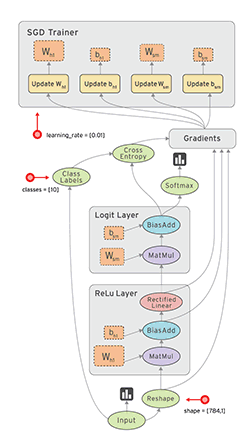TensorFlow 中的数据流图有以下几个优点：

可并行 计算节点之间有明确的线进行连接，系统可以很容易的判断出哪些计算操作可以并行执行
可分发 图中的各个节点可以分布在不同的计算单元(CPU、 GPU、 TPU等)或者不同的机器中，每个节点产生的数据可以通过明确的线发送的下一个节点中
可优化 TensorFlow 中的 XLA 编译器可以根据数据流图进行代码优化，加快运行速度
可移植 数据流图的信息可以不依赖代码进行保存，如使用Python创建的图，经过保存后可以在C++或Java中使用
Sesssion

我们在Python中需要做一些计算操作时一般会使用NumPy，NumPy在做矩阵操作等复杂的计算的时候会使用其他语言(C/C++)来实现这些计算逻辑，来保证计算的高效性。但是频繁的在多个编程语言间切换也会有一定的耗时，如果只是单机操作这些耗时可能会忽略不计，但是如果在分布式并行计算中，计算操作可能分布在不同的CPU、GPU甚至不同的机器中，这些耗时可能会比较严重。
TensorFlow 底层是使用C++实现，这样可以保证计算效率，并使用 tf.Session类来连接客户端程序与C++运行时。上层的Python、Java等代码用来设计、定义模型，构建的Graph，最后通过tf.Session.run()方法传递给底层执行。

构建计算图

上面介绍的是 TensorFlow 和 Graph 的概念，下面介绍怎么用 Tensor 构建 Graph。
Tensor 即可以表示输入、输出的端点，还可以表示计算单元，如下的代码创建了对两个 Tensor 执行 + 操作的 Tensor：

import tensorflow as tf
# 创建两个常量节点
node1 = tf.constant(3.2)
node2 = tf.constant(4.8)
# 创建一个 adder 节点，对上面两个节点执行 + 操作
sess = tf.Session()
print(sess.run(adder))

上面print的输出为：

Tensor("add:0", shape=(), dtype=float32)
8.0

上面使用tf.constant()创建的 Tensor 都是常量，一旦创建后其中的值就不能改变了。有时我们还会需要从外部输入数据，这时可以用tf.placeholder 创建占位 Tensor，占位 Tensor 的值可以在运行的时候输入。如下就是创建占位 Tensor 的例子：

import tensorflow as tf
# 创建两个占位 Tensor 节点
a = tf.placeholder(tf.float32)
b = tf.placeholder(tf.float32)
# 创建一个 adder 节点，对上面两个节点执行 + 操作
# 打印三个节点
print(a)
print(b)
# 运行一下，后面的 dict 参数是为占位 Tensor 提供输入数据
sess = tf.Session()
print(sess.run(adder, {a: [1, 3], b: [2, 4]}))

上面代码的输出为：

Tensor("Placeholder:0", dtype=float32)
Tensor("Placeholder_1:0", dtype=float32)
7.5
[ 3.  7.]

我们还可以添加其他操作构建复杂的 Graph

# 添加×操作
print(sess.run(add_and_triple, {a: 3, b: 4.5}))

上面的输出为

22.5

TensorFlow 应用实例

上面介绍了 TensorFlow 中的一些基本概念，下面我们通过一个小例子来了解一下怎么使用 TensorFlow 进行机器学习。

建立模型(Model)

如下为我们进行某项实验获得的一些实验数据：

输入
输出
1
4.8
2
8.5
3
10.4
6
21
8
25.3
我们将这些数据放到一个二维图上可以看的更直观一些，如下，这些数据在图中表现为一些离散的点：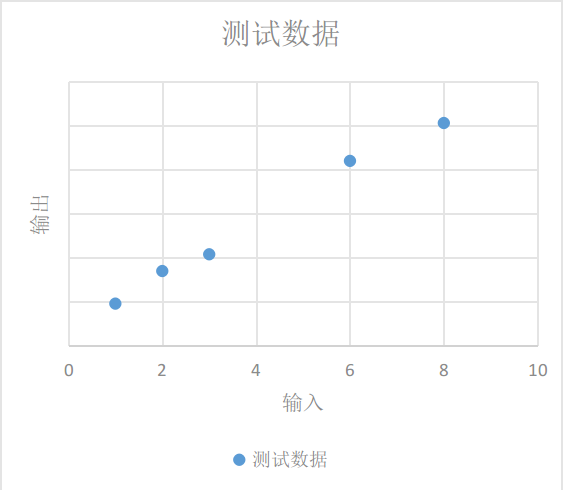我们需要根据现有的这些数据归纳出一个通用模型，通过这个模型我们可以预测其他的输入值产生的输出值。
如下图，我们选择的模型既可以是红线表示的鬼都看不懂的曲线模型，也可以是蓝线表示的线性模型，在概率统计理论的分析中，这两种模型符合真实模型的概率是一样的。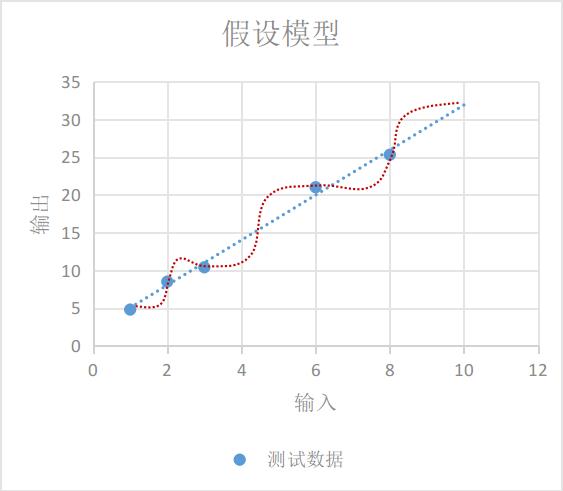根据 “奥卡姆剃刀原则-若有多个假设与观察一致，则选最简单的那个，蓝线表示的线性模型更符合我们的直观预期。
如果用 x<!--//--><![CDATA[//><!--
x
//--><!]]> 表示输入， y<!--//--><![CDATA[//><!--
y
//--><!]]> 表示输出，线性模型可以用下面的方程表示： y=W×x+b<!--//--><![CDATA[//><!--
y = W \times x + b
//--><!]]>
即使我们选择了直线模型，可以选择的模型也会有很多，如下图的三条直线都像是一种比较合理的模型，只是W<!--//--><![CDATA[//><!--
W
//--><!]]>和b<!--//--><![CDATA[//><!--
b
//--><!]]>参数不同。这时我们需要设计一个损失模型(loss model)，来评估一下哪个模型更合理一些，并找到一个最准确的模型。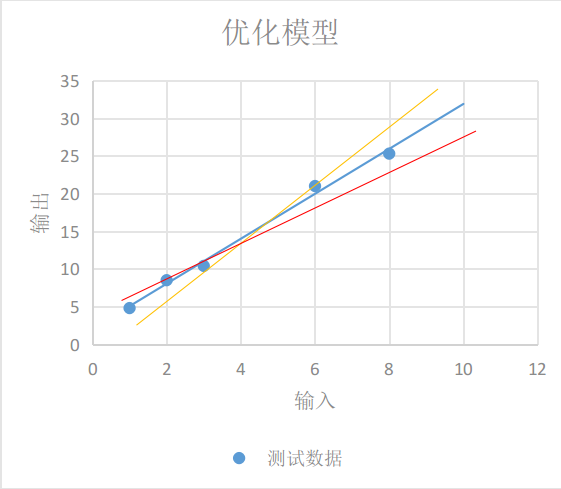如下图每条黄线代表线性模型计算出来的值与实际输出值之间的差值: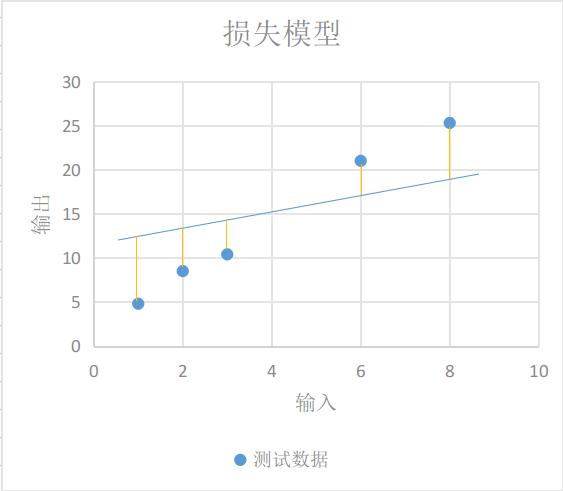我们用y′<!--//--><![CDATA[//><!--
y'
//--><!]]>表示实验得到的实际输出，用下面的方程表示我们的损失模型： loss=∑n=1N(yn−y′n)2<!--//--><![CDATA[//><!--
loss = \sum_{n=1}^N(y_n - y'_n)^2
//--><!]]>显然，损失模型里得到的loss<!--//--><![CDATA[//><!--
loss
//--><!]]>越小，说明我们的线性模型越准确。

使用 TensorFlow 实现模型

上面我们根据实验数据建立了一个线性模型，并为这个线性模型设计了一个损失模型，下面介绍的是怎么在 TensorFlow 中实现我们设计的模型。
在我们的线性模型 y=W×x+b<!--//--><![CDATA[//><!--
y=W \times x + b
//--><!]]> 中，输入x<!--//--><![CDATA[//><!--
x
//--><!]]>可以用占位 Tensor 表示，输出y<!--//--><![CDATA[//><!--
y
//--><!]]>可以用线性模型的输出表示，我们需要不断的改变W<!--//--><![CDATA[//><!--
W
//--><!]]>和b<!--//--><![CDATA[//><!--
b
//--><!]]>的值，来找到一个使loss<!--//--><![CDATA[//><!--
loss
//--><!]]>最小的值。这里W<!--//--><![CDATA[//><!--
W
//--><!]]>和b<!--//--><![CDATA[//><!--
b
//--><!]]>可以用变量 Tensor 表示。使用tf.Variable()可以创建一个变量 Tensor，如下就是我们模型的实现代码：

import tensorflow as tf

# 创建变量 W 和 b 节点，并设置初始值
W = tf.Variable([.1], dtype=tf.float32)
b = tf.Variable([-.1], dtype=tf.float32)
# 创建 x 节点，用来输入实验中的输入数据
x = tf.placeholder(tf.float32)
# 创建线性模型
linear_model = W*x + b

# 创建 y 节点，用来输入实验中得到的输出数据，用于损失模型计算
y = tf.placeholder(tf.float32)
# 创建损失模型
loss = tf.reduce_sum(tf.square(linear_model - y))

# 创建 Session 用来计算模型
sess = tf.Session()

通过tf.Variable()创建变量 Tensor 时需要设置一个初始值，但这个初始值并不能立即使用，例如上面的代码中，我们使用print(sess.run(W))尝试打印W的值会得到下面提示未初始化的异常

tensorflow.python.framework.errors_impl.FailedPreconditionError: Attempting to use uninitialized value Variable

变量 Tensor 需要经过下面的 init  过程后才能使用：

# 初始化变量
init = tf.global_variables_initializer()
sess.run(init)

这之后再使用print(sess.run(W))打印就可以看到我们之前赋的初始值：

[ 0.1]

变量初始化完之后，我们可以先用上面对W和b设置的初始值0.1和-0.1运行一下我们的线性模型看看结果：

print(sess.run(linear_model, {x: [1, 2, 3, 6, 8]}))

输出结果为：

[ 0.          0.1         0.20000002  0.5         0.69999999]

貌似与我们实验的实际输出差距很大，我们再运行一下损失模型：

print(sess.run(loss, {x: [1, 2, 3, 6, 8], y: [4.8, 8.5, 10.4, 21.0, 25.3]}))

得到的损失值也很大

1223.05

我们可以用tf.assign()对W和b变量重新赋值再检验一下：

# 给 W 和 b 赋新值
fixW = tf.assign(W, [2.])
fixb = tf.assign(b, [1.])
# run 之后新值才会生效
sess.run([fixW, fixb])
# 重新验证损失值
print(sess.run(loss, {x: [1, 2, 3, 6, 8], y: [4.8, 8.5, 10.4, 21.0, 25.3]}))

输出的损失值比之前的小了很多：

159.94

我们需要不断调整变量W和b的值，找到使损失值最小的W和b。这肯定是一个very boring的过程，因此 TensorFlow 提供了训练模型的方法，自动帮我们进行这些繁琐的训练工作。

使用 TensorFlow 训练模型

如下的代码就是使用梯度下降优化算法帮助我们训练模型：

# 创建一个梯度下降优化器，学习率为0.001
train = optimizer.minimize(loss)

# 用两个数组保存训练数据
x_train = [1, 2, 3, 6, 8]
y_train = [4.8, 8.5, 10.4, 21.0, 25.3]

# 训练10000次
for i in range(10000):
sess.run(train, {x: x_train, y: y_train})

# 打印一下训练后的结果
print('W: %s b: %s loss: %s' % (sess.run(W), sess.run(b), sess.run(loss, {x: x_train , y: y_train})))

打印出来的训练结果如下，可以看到损失值已经很小了：

W: [ 2.98236108] b: [ 2.07054377] loss: 2.12941

我们整理一下前面的代码，完整的demo代码如下，将下面的代码保存在一个demo.py文件里，通过python3 demo.py执行一下就可以看到训练结果了：

import tensorflow as tf

# 创建变量 W 和 b 节点，并设置初始值
W = tf.Variable([.1], dtype=tf.float32)
b = tf.Variable([-.1], dtype=tf.float32)
# 创建 x 节点，用来输入实验中的输入数据
x = tf.placeholder(tf.float32)
# 创建线性模型
linear_model = W * x + b

# 创建 y 节点，用来输入实验中得到的输出数据，用于损失模型计算
y = tf.placeholder(tf.float32)
# 创建损失模型
loss = tf.reduce_sum(tf.square(linear_model - y))

# 创建 Session 用来计算模型
sess = tf.Session()

# 初始化变量
init = tf.global_variables_initializer()
sess.run(init)

# 创建一个梯度下降优化器，学习率为0.001
train = optimizer.minimize(loss)

# 用两个数组保存训练数据
x_train = [1, 2, 3, 6, 8]
y_train = [4.8, 8.5, 10.4, 21.0, 25.3]

# 训练10000次
for i in range(10000):
sess.run(train, {x: x_train, y: y_train})

# 打印一下训练后的结果
print('W: %s b: %s loss: %s' % (sess.run(W), sess.run(
b), sess.run(loss, {x: x_train, y: y_train})))

TensorFlow 高级训练模型

tf.estimator是TensorFlow提供的高级库，提供了很多常用的训练模型，可以简化机器学习中的很多训练过程，如：

运行训练循环
运行评估循环
管理训练数据集
评估模型

前面的demo中我们构建了一个线性模型，通过使用一组实验数据训练我们的线性模型，我们得到了一个自认为损失最小的最优模型，根据训练结果我们的最优模型可以表示为下面的方程： y=2.98x+2.07<!--//--><![CDATA[//><!--
y = 2.98x + 2.07
//--><!]]>
但是这个我们自认为的最优模型是否会一直是最优的？我们需要通过一些新的实验数据来评估(evaluation)模型的泛化性能(generalization performance)，如果新的实验数据应用到到这个模型中损失值越小，那么这个模型的泛化性能就越好，反之就越差。下面的demo中我们也会看到怎么评估模型。

使用LinearRegressor

前面我们构建了一个线性模型，通过训练得到一个线性回归方程。tf.estimator中也提供了线性回归的训练模型tf.estimator.LinearRegressor，下面的代码就是使用LinearRegressor训练并评估模型的方法：

# 我们会用到NumPy来处理各种训练数据
import numpy as np
import tensorflow as tf

# 创建一个特征向量列表，该特征列表里只有一个特征向量，
# 该特征向量为实数向量，只有一个元素的数组，且该元素名称为 x，
# 我们还可以创建其他更加复杂的特征列表
feature_columns = [tf.feature_column.numeric_column("x", shape=)]

# 创建一个LinearRegressor训练器，并传入特征向量列表
estimator = tf.estimator.LinearRegressor(feature_columns=feature_columns)

# 保存训练用的数据
x_train = np.array([1., 2., 3., 6., 8.])
y_train = np.array([4.8, 8.5, 10.4, 21.0, 25.3])

# 保存评估用的数据
x_eavl = np.array([2., 5., 7., 9.])
y_eavl = np.array([7.6, 17.2, 23.6, 28.8])

# 用训练数据创建一个输入模型，用来进行后面的模型训练
# 第一个参数用来作为线性回归模型的输入数据
# 第二个参数用来作为线性回归模型损失模型的输入
# 第三个参数batch_size表示每批训练数据的个数
# 第四个参数num_epochs为epoch的次数，将训练集的所有数据都训练一遍为1次epoch
# 低五个参数shuffle为取训练数据是顺序取还是随机取
train_input_fn = tf.estimator.inputs.numpy_input_fn(
{"x": x_train}, y_train, batch_size=2, num_epochs=None, shuffle=True)

# 再用训练数据创建一个输入模型，用来进行后面的模型评估
train_input_fn_2 = tf.estimator.inputs.numpy_input_fn(
{"x": x_train}, y_train, batch_size=2, num_epochs=1000, shuffle=False)

# 用评估数据创建一个输入模型，用来进行后面的模型评估
eval_input_fn = tf.estimator.inputs.numpy_input_fn(
{"x": x_eavl}, y_eavl, batch_size=2, num_epochs=1000, shuffle=False)

# 使用训练数据训练1000次
estimator.train(input_fn=train_input_fn, steps=1000)

# 使用原来训练数据评估一下模型，目的是查看训练的结果
train_metrics = estimator.evaluate(input_fn=train_input_fn_2)
print("train metrics: %r" % train_metrics)

# 使用评估数据评估一下模型，目的是验证模型的泛化性能
eval_metrics = estimator.evaluate(input_fn=eval_input_fn)
print("eval metrics: %s" % eval_metrics)

运行上面的代码输出为：

train metrics: {'loss': 1.0493528, 'average_loss': 0.52467638, 'global_step': 1000}
eval metrics: {'loss': 0.72186172, 'average_loss': 0.36093086, 'global_step': 1000}

评估数据的loss比训练数据还要小，说明我们的模型泛化性能很好。

自定义Estimator模型

tf.estimator库中提供了很多预定义的训练模型，但是有可能这些训练模型不能满足我们的需求，我们需要使用自己构建的模型。
我们可以通过实现tf.estimator.Estimator的子类来构建我们自己的训练模型，LinearRegressor就是Estimator的一个子类。另外我们也可以只给Estimator基类提供一个model_fn的实现，定义我们自己的模型训练、评估方法以及计算损失的方法。
下面的代码就是使用我们最开始构建的线性模型实现自定义Estimator的实例。

import numpy as np
import tensorflow as tf

# 定义模型训练函数，同时也定义了特征向量

def model_fn(features, labels, mode):
# 构建线性模型
W = tf.get_variable("W", , dtype=tf.float64)
b = tf.get_variable("b", , dtype=tf.float64)
y = W * features['x'] + b
# 构建损失模型
loss = tf.reduce_sum(tf.square(y - labels))
# 训练模型子图
global_step = tf.train.get_global_step()
train = tf.group(optimizer.minimize(loss),
# 通过EstimatorSpec指定我们的训练子图积极损失模型
return tf.estimator.EstimatorSpec(
mode=mode,
predictions=y,
loss=loss,
train_op=train)

# 创建自定义的训练模型
estimator = tf.estimator.Estimator(model_fn=model_fn)

# 后面的训练逻辑与使用LinearRegressor一样
x_train = np.array([1., 2., 3., 6., 8.])
y_train = np.array([4.8, 8.5, 10.4, 21.0, 25.3])

x_eavl = np.array([2., 5., 7., 9.])
y_eavl = np.array([7.6, 17.2, 23.6, 28.8])

train_input_fn = tf.estimator.inputs.numpy_input_fn(
{"x": x_train}, y_train, batch_size=2, num_epochs=None, shuffle=True)

train_input_fn_2 = tf.estimator.inputs.numpy_input_fn(
{"x": x_train}, y_train, batch_size=2, num_epochs=1000, shuffle=False)

eval_input_fn = tf.estimator.inputs.numpy_input_fn(
{"x": x_eavl}, y_eavl, batch_size=2, num_epochs=1000, shuffle=False)

estimator.train(input_fn=train_input_fn, steps=1000)

train_metrics = estimator.evaluate(input_fn=train_input_fn_2)
print("train metrics: %r" % train_metrics)

eval_metrics = estimator.evaluate(input_fn=eval_input_fn)
print("eval metrics: %s" % eval_metrics)

上面代码的输出为

train metrics: {'loss': 0.8984344, 'global_step': 1000}
eval metrics: {'loss': 0.48776609, 'global_step': 1000}

TensorBoard

为了更方便 TensorFlow 的建模和调优，Google 还为 TensorFlow 开发了一款可视化的工具：TensorBoard，将我们第一个Demo的代码稍微改造一下，就可以使用 TensorBoard更加直观的理解 TensorFlow 的训练过程。

import tensorflow as tf

# 创建节点时设置name，方便在图中识别
W = tf.Variable(, dtype=tf.float32, name='W')
b = tf.Variable(, dtype=tf.float32, name='b')

# 创建节点时设置name，方便在图中识别
x = tf.placeholder(tf.float32, name='x')
y = tf.placeholder(tf.float32, name='y')

# 线性模型
linear_model = W * x + b

# 损失模型隐藏到loss-model模块
with tf.name_scope("loss-model"):
loss = tf.reduce_sum(tf.square(linear_model - y))
# 给损失模型的输出添加scalar，用来观察loss的收敛曲线
tf.summary.scalar("loss", loss)

train = optmizer.minimize(loss)

x_train = [1, 2, 3, 6, 8]
y_train = [4.8, 8.5, 10.4, 21.0, 25.3]

sess = tf.Session()
init = tf.global_variables_initializer()
sess.run(init)

# 调用 merge_all() 收集所有的操作数据
merged = tf.summary.merge_all()

# 模型运行产生的所有数据保存到 /tmp/tensorflow 文件夹供 TensorBoard 使用
writer = tf.summary.FileWriter('/tmp/tensorflow', sess.graph)

# 训练10000次
for i in range(10000):
# 训练时传入merge
summary, _ = sess.run([merged, train], {x: x_train, y: y_train})
# 收集每次训练产生的数据

curr_W, curr_b, curr_loss = sess.run(
[W, b, loss], {x: x_train, y: y_train})

print("After train W: %s b %s loss: %s" % (curr_W, curr_b, curr_loss))

运行完上面的代码后，训练过程产生的数据就保存在 /tmp/tensorflow 文件夹了，我们可以在命令行终端运行下面的命令启动 TensorBoard：

# 通过 --logdir 参数设置我们存放训练数据的目录
\$ tensorboard --logdir /tmp/tensorflow

然后在浏览器中打开 http://localhost:6006 页面就可以看到我们的模型数据了。
首先在 SCALARS 页面我们可以看到我们通过 tf.summary.scalar("loss", loss)设置的loss收敛曲线，从曲线图中可以看出在训练了大概2000次的时候loss就已经收敛的差不多了。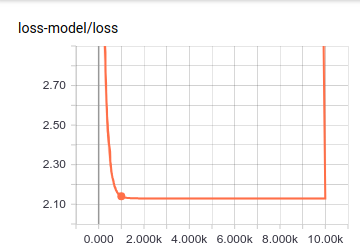在 GRAPHS 页面可以看到我们构建的模型的数据流图：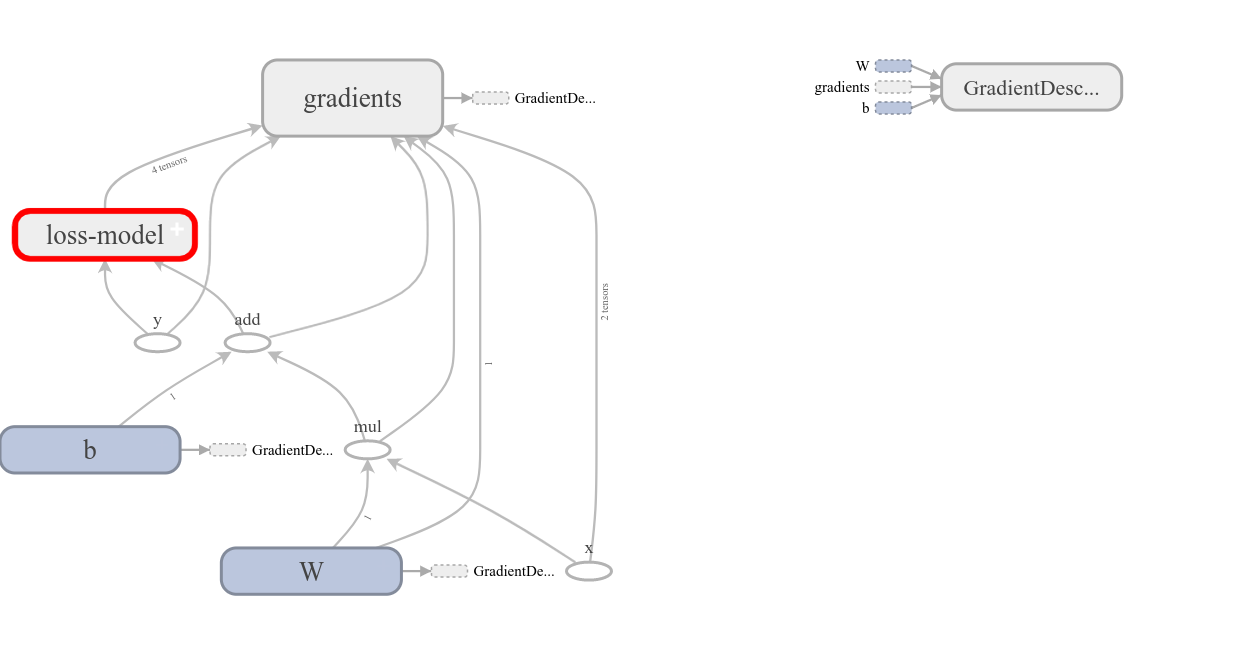其中损失模型折叠到loss-model模块里了，双击该模块可以展开损失模型的内容：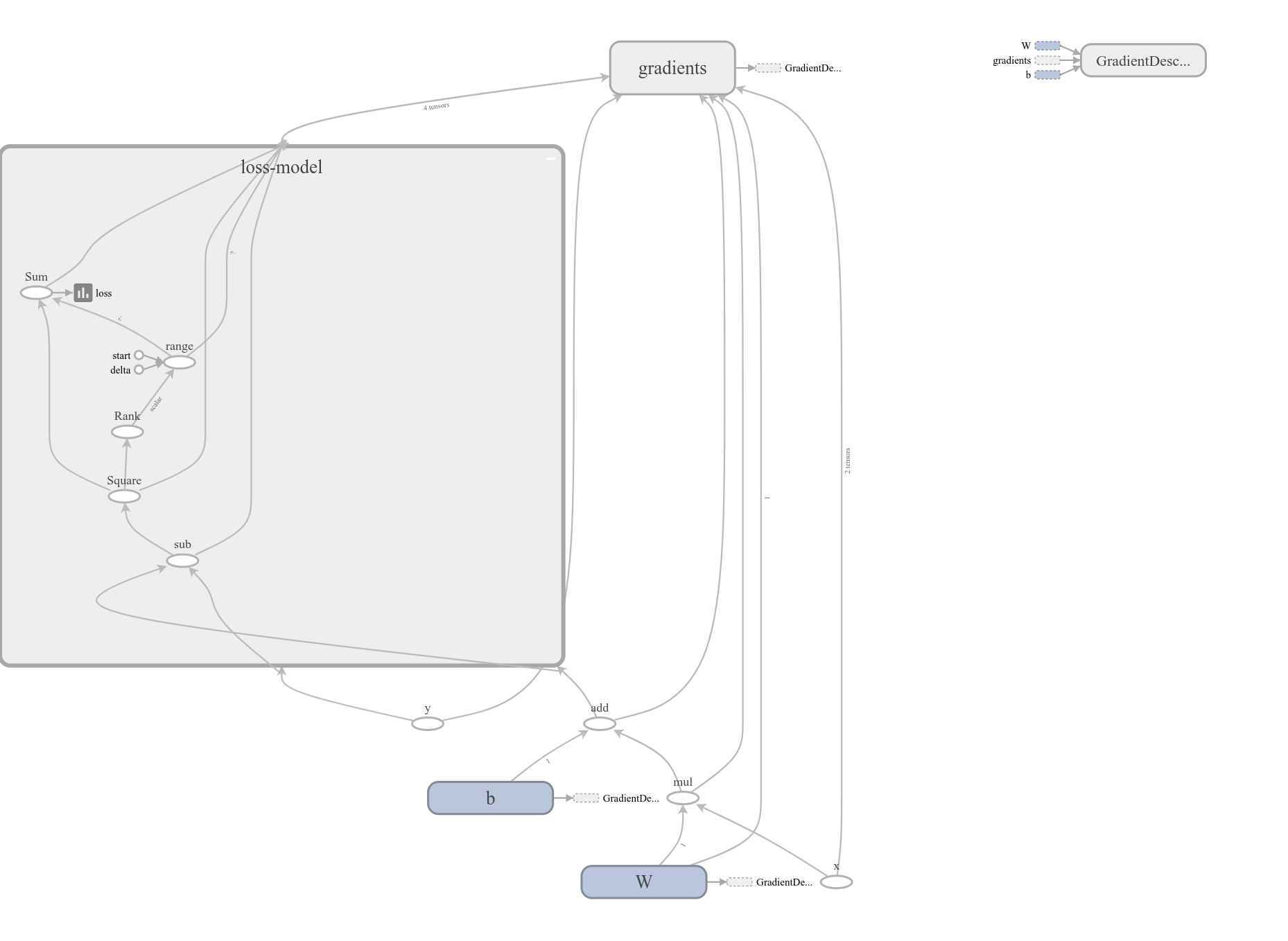– end –

如上即为本人初学 TensorFlow 时的入门 demo，如果想对 TensorFlow 有更深的了解可以参考如下网站：

官方网站
官方中文网站
国内爱好者翻译的中文文档

展开全文机器学习 python
• 作者：叶 虎 编辑：陈人和 前 言TensorFlow虽是深度学习领域最广泛使用的框架，但是对比PyTorch这一动态...作者：叶  虎            编辑：陈人和           前  言TensorFlow虽是深度学习领域最广泛使用的框架，但是对比PyTorch这一动态图框架，采用静态图（Graph模式）的TensorFlow确实是难用。好在最近TensorFlow支持了eager模式，对标PyTorch的动态执行机制。更进一步地，Google在最近推出了全新的版本TensorFlow 2.0，2.0版本相比1.0版本不是简单地更新，而是一次重大升级（虽然目前只发布了preview版本）。简单地来说，TensorFlow 2.0默认采用eager执行模式，而且重整了很多混乱的模块。毫无疑问，2.0版本将会逐渐替换1.0版本，所以很有必要趁早入手TensorFlow 2.0。这篇文章将简明扼要地介绍TensorFlow 2.0，以求快速入门。章节目录Eager执行AutoGraph性能优化：tf.function01Eager执行TensorFlow的Eager执行时一种命令式编程（imperative programming），这和原生Python是一致的，当你执行某个操作时是立即返回结果的。而TensorFlow一直是采用Graph模式，即先构建一个计算图，然后需要开启Session，喂进实际的数据才真正执行得到结果。显然，eager执行更简洁，我们可以更容易debug自己的代码，这也是为什么PyTorch更简单好用的原因。一个简单的例子如下：x = tf.ones((2, 2), dtype=tf.dtypes.float32)
y = tf.constant([[1, 2],
[3, 4]], dtype=tf.dtypes.float32)
z = tf.matmul(x, y)
print(z)
# tf.Tensor(
# [[4. 6.]
#  [4. 6.]], shape=(2, 2), dtype=float32)
print(z.numpy())
# [[4. 6.]
# [4. 6.]]可以看到在eager执行下，每个操作后的返回值是tf.Tensor，其包含具体值，不再像Graph模式下那样只是一个计算图节点的符号句柄。由于可以立即看到结果，这非常有助于程序debug。更进一步地，调用tf.Tensor.numpy()方法可以获得Tensor所对应的numpy数组。这种eager执行的另外一个好处是可以使用Python原生功能，比如下面的条件判断：random_value = tf.random.uniform([], 0, 1)
x = tf.reshape(tf.range(0, 4), [2, 2])
print(random_value)
if random_value.numpy() > 0.5:
y = tf.matmul(x, x)
else:
y = tf.add(x, x)这种动态控制流主要得益于eager执行得到Tensor可以取出numpy值，这避免了使用Graph模式下的tf.cond和tf.while等算子。另外一个重要的问题，在egaer模式下如何计算梯度。在Graph模式时，我们在构建模型前向图时，同时也会构建梯度图，这样实际喂数据执行时可以很方便计算梯度。但是eager执行是动态的，这就需要每一次执行都要记录这些操作以计算梯度，这是通过tf.GradientTape来追踪所执行的操作以计算梯度，下面是一个计算实例：w = tf.Variable([[1.0]])
loss = w * w + 2. * w + 5.
print(grad)  # => tf.Tensor([[ 4.]], shape=(1, 1), dtype=float32)对于eager执行，每个tape会记录当前所执行的操作，这个tape只对当前计算有效，并计算相应的梯度。PyTorch也是动态图模式，但是与TensorFlow不同，它是每个需要计算Tensor会拥有grad_fn以追踪历史操作的梯度。TensorFlow 2.0引入的eager提高了代码的简洁性，而且更容易debug。但是对于性能来说，eager执行相比Graph模式会有一定的损失。这不难理解，毕竟原生的Graph模式是先构建好静态图，然后才真正执行。这对于在分布式训练、性能优化和生产部署方面具有优势。但是好在，TensorFlow 2.0引入了tf.function和AutoGraph来缩小eager执行和Graph模式的性能差距，其核心是将一系列的Python语法转化为高性能的graph操作。02AutoGraphAutoGraph在TensorFlow 1.x已经推出，主要是可以将一些常用的Python代码转化为TensorFlow支持的Graph代码。一个典型的例子是在TensorFlow中我们必须使用tf.while和tf.cond等复杂的算子来实现动态流程控制，但是现在我们可以使用Python原生的for和if等语法写代码，然后采用AutoGraph转化为TensorFlow所支持的代码，如下面的例子:def square_if_positive(x):
if x > 0:
x = x * x
else:
x = 0.0
return x
# eager 模式
print('Eager results: %2.2f, %2.2f' % (square_if_positive(tf.constant(9.0)),
square_if_positive(tf.constant(-9.0))))
# graph 模式
tf_square_if_positive = tf.autograph.to_graph(square_if_positive)
with tf.Graph().as_default():
# The result works like a regular op: takes tensors in, returns tensors.
# You can inspect the graph using tf.get_default_graph().as_graph_def()
g_out1 = tf_square_if_positive(tf.constant( 9.0))
g_out2 = tf_square_if_positive(tf.constant(-9.0))
with tf.compat.v1.Session() as sess:
print('Graph results: %2.2f, %2.2f\n' % (sess.run(g_out1), sess.run(g_out2)))上面我们定义了一个square_if_positive函数，它内部使用的Python的原生的if语法，对于TensorFlow 2.0的eager执行，这是没有问题的。然而这是TensorFlow 1.x所不支持的，但是使用AutoGraph可以将这个函数转为Graph函数，你可以将其看成一个常规TensorFlow op，其可以在Graph模式下运行（tf2 没有Session，这是tf1.x的特性，想使用tf1.x的话需要调用tf.compat.v1）。大家要注意eager模式和Graph模式的差异，尽管结果是一样的，但是Graph模式更高效。 从本质上讲，AutoGraph是将Python代码转为TensorFlow原生的代码，我们可以进一步看到转化后的代码:print(tf.autograph.to_code(square_if_positive))
#################################################
from __future__ import print_function
def tf__square_if_positive(x):
try:
with ag__.function_scope('square_if_positive'):
do_return = False
retval_ = None
cond = ag__.gt(x, 0)
def if_true():
with ag__.function_scope('if_true'):
x_1, = x,
x_1 = x_1 * x_1
return x_1
def if_false():
with ag__.function_scope('if_false'):
x = 0.0
return x
x = ag__.if_stmt(cond, if_true, if_false)
do_return = True
retval_ = x
return retval_
except:
ag__.rewrite_graph_construction_error(ag_source_map__)
tf__square_if_positive.autograph_info__ = {}可以看到AutoGraph转化的代码定义了两个条件函数，然后调用if_stmt op，应该就是类似tf.cond的op。AutoGraph支持很多Python特性，比如循环: def sum_even(items):
s = 0
for c in items:
if c % 2 > 0:
continue
s += c
return s
print('Eager result: %d' % sum_even(tf.constant([10,12,15,20])))
tf_sum_even = tf.autograph.to_graph(sum_even)
with tf.Graph().as_default(), tf.compat.v1.Session() as sess:
print('Graph result: %d\n\n' % sess.run(tf_sum_even(tf.constant([10,12,15,20]))))对于大部分Python特性AutoGraph是支持的，但是其仍然有限制，具体可以见Capabilities and Limitations。链接：https://github.com/tensorflow/tensorflow/blob/master/tensorflow/python/autograph/LIMITATIONS.md此外，要注意的一点是，经过AutoGraph转换的新函数是可以eager模式下执行的，但是性能却并不会比转换前的高，你可以对比：x = tf.constant([10, 12, 15, 20])
print("Eager at orginal code:", timeit.timeit(lambda: sum_even(x), number=100))
print("Eager at autograph code:", timeit.timeit(lambda: tf_sum_even(x), number=100))
with tf.Graph().as_default(), tf.compat.v1.Session() as sess:
graph_op = tf_sum_even(tf.constant([10, 12, 15, 20]))
sess.run(graph_op)  # remove first call
print("Graph at autograph code:", timeit.timeit(lambda: sess.run(graph_op), number=100))
##########################################
Eager at orginal code: 0.05176109499999981
Eager at autograph code: 0.11203173799999977
Graph at autograph code: 0.03418808900000059从结果上看，Graph模式下的执行效率是最高的，原来的代码在eager模式下效率次之，经AutoGraph转换后的代码效率最低。所以，在TensorFlow 2.0，我们一般不会直接使用tf.autograph，因为eager执行下效率没有提升。要真正达到Graph模式下的效率，要依赖tf.function这个更强大的利器。03性能优化：tf.function条件随机场（Conditional Random Field，简称CRF）是一种判别式无向图模型。生成式模型是直接对联合分布进行建模，而判别式模型则是对条件分布进行建模。前面介绍的隐马尔可夫模型和马尔可夫随机场都是生成式模型，而条件随机场是判别式模型。尽管eager执行更简洁，但是Graph模式却是性能更高，为了减少这个性能gap，TensorFlow 2.0引入了tf.function，先给出官方对tf.function的说明:function constructs a callable that executes a TensorFlow graph (tf.Graph) created by tracing the TensorFlow operations in func. This allows the TensorFlow runtime to apply optimizations and exploit parallelism in the computation defined by func.简单来说，就是tf.function可以将一个func中的TensorFlow操作构建为一个Graph，这样在调用时是执行这个Graph，这样计算性能更优。比如下面的例子:def f(x, y):
print(x, y)
return tf.reduce_mean(tf.multiply(x ** 2, 3) + y)
g = tf.function(f)
x = tf.constant([[2.0, 3.0]])
y = tf.constant([[3.0, -2.0]])
# f and g will return the same value, but g will be executed as a
# TensorFlow graph.
assert f(x, y).numpy() == g(x, y).numpy()
# tf.Tensor([[2. 3.]], shape=(1, 2), dtype=float32) tf.Tensor([[ 3. -2.]], shape=(1, 2), dtype=float32)
# Tensor("x:0", shape=(1, 2), dtype=float32) Tensor("y:0", shape=(1, 2), dtype=float32)如上面的例子，被tf.function装饰的函数将以Graph模式执行，可以把它想象一个封装了Graph的TF op，直接调用它也会立即得到Tensor结果，但是其内部是高效执行的。我们在内部打印Tensor时，eager执行会直接打印Tensor的值，而Graph模式打印的是Tensor句柄，其无法调用numpy方法取出值，这和TF 1.x的Graph模式是一致的。由于tf.function装饰的函数是Graph执行，其执行速度一般要比eager模式要快，当Graph包含很多小操作时差距更明显，可以比较下卷积和LSTM的性能差距：import timeit
conv_layer = tf.keras.layers.Conv2D(100, 3)
@tf.function
def conv_fn(image):
return conv_layer(image)
image = tf.zeros([1, 200, 200, 100])
# warm up
conv_layer(image); conv_fn(image)
print("Eager conv:", timeit.timeit(lambda: conv_layer(image), number=10))
print("Function conv:", timeit.timeit(lambda: conv_fn(image), number=10))
# 单纯的卷积差距不是很大
# Eager conv: 0.44013839924952197
# Function conv: 0.3700763391782858
lstm_cell = tf.keras.layers.LSTMCell(10)
@tf.function
def lstm_fn(input, state):
return lstm_cell(input, state)
input = tf.zeros([10, 10])
state = [tf.zeros([10, 10])] * 2
# warm up
lstm_cell(input, state); lstm_fn(input, state)
print("eager lstm:", timeit.timeit(lambda: lstm_cell(input, state), number=10))
print("function lstm:", timeit.timeit(lambda: lstm_fn(input, state), number=10))
# 对于LSTM比较heavy的计算，Graph执行要快很多
# eager lstm: 0.025562446062237565
# function lstm: 0.0035498656569271647要想灵活使用tf.function，必须深入理解它背后的机理，这里简单地谈一下。在TF 1.x时，首先要创建静态计算图，然后新建Session真正执行不同的运算：import tensorflow as tf
x = tf.placeholder(tf.float32)
y = tf.square(x)
sess = tf.Session()
z0 = sess.run([z], feed_dict={x: 2.})        # 6.0
z1 = sess.run([z], feed_dict={x: 2., y: 2.}) # 4.0尽管上面只定义了一个graph，但是两次不同的sess执行（运行时）其实是执行两个不同的程序或者说subgraph：def compute_z0(x):
def compute_z1(x, y):
return tf.add(x,  y)这里我们将两个不同的subgraph封装到了两个python函数中。更进一步地，我们可以不再需要Session，当执行这两个函数时，直接调用对应的计算图就可以，这就是tf.function的功效：import tensorflow as tf
@tf.function
def compute_z1(x, y):
@tf.function
def compute_z0(x):
return compute_z1(x, tf.square(x))
z0 = compute_z0(2.)
z1 = compute_z1(2., 2.)可以说tf.function内部管理了一系列Graph，并控制了Graph的执行。另外一个问题时，虽然函数内部定义了一系列的操作，但是对于不同的输入，是需要不同的计算图。如函数的输入Tensor的shape或者dtype不同，那么计算图是不同的，好在tf.function支持这种多态性（polymorphism）# Functions are polymorphic
@tf.function
def double(a):
print("Tracing with", a)
return a + a
print(double(tf.constant(1)))
print(double(tf.constant(1.1)))
print(double(tf.constant([1, 2])))
# Tracing with Tensor("a:0", shape=(), dtype=int32)
# tf.Tensor(2, shape=(), dtype=int32)
# Tracing with Tensor("a:0", shape=(), dtype=float32)
# tf.Tensor(2.2, shape=(), dtype=float32)
# Tracing with Tensor("a:0", shape=(2,), dtype=int32)
# tf.Tensor([2 4], shape=(2,), dtype=int32)注意函数内部的打印，当输入tensor的shape或者类型发生变化，打印的东西也是相应改变。所以，它们的计算图（静态的）并不一样。tf.function这种多态特性其实是背后追踪了（tracing）不同的计算图。具体来说，被tf.function装饰的函数f接受一定的Tensors，并返回0到任意到Tensor，当装饰后的函数F被执行时：根据输入Tensors的shape和dtypes确定一个"trace_cache_key"；每个"trace_cache_key"映射了一个Graph，当新的"trace_cache_key"要建立时，f将构建一个新的Graph，若"trace_cache_key"已经存在，那么直需要从缓存中查找已有的Graph即可；将输入Tensors喂进这个Graph，然后执行得到输出Tensors。这种多态性是我们需要的，因为有时候我们希望输入不同shape或者dtype的Tensors，但是当"trace_cache_key"越来越多时，意味着你要cache了庞大的Graph，这点是要注意的。另外，tf.function提供了input_signature，这个参数采用tf.TensorSpec指定了输入到函数的Tensor的shape和dtypes，如下面的例子：@tf.function(input_signature=[tf.TensorSpec(shape=None, dtype=tf.float32)])
def f(x):
print(f(tf.constant(1.0)))  # tf.Tensor(2.0, shape=(), dtype=float32)
print(f(tf.constant([1.0,]))) # tf.Tensor([2.], shape=(1,), dtype=float32)
print(f(tf.constant()))  # ValueError: Python inputs incompatible with input_signature此时，输入Tensor的dtype必须是float32，但是shape不限制，当类型不匹配时会出错。tf.function的另外一个参数是autograph，默认是True，意思是在构建Graph时将自动使用AutoGraph，这样你可以在函数内部使用Python原生的条件判断以及循环语句，因为它们会被tf.cond和tf.while_loop转化为Graph代码。注意的一点是判断分支和循环必须依赖于Tensors才会被转化，当autograph为False时，如果存在判断分支和循环必须依赖于Tensors的情况将会出错。如下面的例子。def sum_even(items):
s = 0
for c in items:
if c % 2 > 0:
continue
s += c
return s
sum_even_autograph_on = tf.function(sum_even, autograph=True)
sum_even_autograph_off = tf.function(sum_even, autograph=False)
x = tf.constant([10, 12, 15, 20])
sum_even(x) # OK
sum_even_autograph_on(x) # OK
sum_even_autograph_off(x) # TypeError: Tensor objects are only iterable when eager execution is enabled很容易理解，应用tf.function之后是Graph模式，Tensors是不能被遍历的，但是采用AutoGraph可以将其转换为Graph代码，所以可以成功。大部分情况，我们还是默认开启autograph。最要的是tf.function可以应用到类方法中，并且可以引用tf.Variable，可以看下面的例子：class ScalarModel(object):
def __init__(self):
self.v = tf.Variable(0)
@tf.function
def increment(self, amount):
model1 = ScalarModel()
model1.increment(tf.constant(3))
assert int(model1.v) == 3
model1.increment(tf.constant(4))
assert int(model1.v) == 7
model2 = ScalarModel()  # model1和model2 拥有不同变量
model2.increment(tf.constant(5))
assert int(model2.v) == 5后面会讲到，这个特性可以应用到tf.Keras的模型构建中。上面这个例子还有一点，就是可以在function中使用tf.assign这类具有副作用（改变Variable的值）的操作，这对于模型训练比较重要。前面说过，python原生的print函数只会在构建Graph时打印一次Tensor句柄。如果想要打印Tensor的具体值，要使用tf.print：@tf.function
def print_element(items):
for c in items:
tf.print(c)
x = tf.constant([1, 5, 6, 8, 3])
print_element(x)这里就对tf.function做这些介绍，但是实际上其还有更多复杂的使用须知，详情可以参考TensorFlow 2.0: Functions, not Sessions。链接：https://github.com/tensorflow/community/blob/master/rfcs/20180918-functions-not-sessions-20.md参考：TensorFlow官网：https://tensorflow.google.cn/versions/r2.0/api_docs/END往期回顾【1】network sliming:加快模型速度同时不损失精度【2】理解Batch Normalization（含实现代码）【3】图像降维之Isomap特征抽取方法【4】语义分割网络经典：FCN与SegNet【5】One Day One GAN [DAY 4]机器学习算法工程师                            一个用心的公众号长按，识别，加关注进群，学习，得帮助你的关注，我们的热度，我们一定给你学习最大的帮助你点的每个赞，我都认真当成了喜欢


展开全文• ## TensorFlow是什么

万次阅读 多人点赞 2018-11-17 22:14:33
1， 谷歌大神带你十分钟看懂TensorFlow 2 你真的懂TensorFlow吗？Tensor是神马？为什么还会Flow？   也许你已经下载了TensorFlow，而且准备开始着手研究深度学习。但是你会疑惑：TensorFlow里面的Tensor，也...
• 快速安装 你可以使用pip安装Rasa和Rasa X(Python版本要求3.5.4及以上) ... 安装有问题？查看分步安装手册 你也可以从源代码编译安装Rasa 从源代码安装Rasa的高级选项和自定义管道的安装说明，请转到这里 ...
• tensorflow 版本提示错误:AttributeError: module 'tensorflow_core._api.v2.train' has no attribute 将 tensorflow 版本降级 2.0换成1.5 pip3 install tensorflow ==1.5版本降级 AttributeError: module ...
• 基本概念 Rasa 是一个基于机器学习实现多轮对话的开源机器人框架，其中包含两个模块Rasa_core：github与Rasa_nlu：github Rasa-Nlu 是自然语言理解模型集合，主要包括意图识别，实体识别，它会把用户的输入转换为...
• 1.No module named ‘rasa_nlu.test’ 解决办法pip install rasa-core[tensorflow]
• 本文讲解在python3.6下安装rasa_core，安装rasa_core不用再单独安装rasa_nlu。 　首先要确保安装了gcc,g++,python3,pip本文不再详解,在下面的安装过程中如果提示少了哪些东西，安装上就可以了 ...
• ## TensorFlow框架

万次阅读 2018-10-24 08:58:27
基于TensorFlow的神经网络：用张量表示数据，用计算图搭建神经网络，用会话执行计算图，优化线上的权重（参数），得到模型。 张量(tensor)：多维数组（列表） 阶：张量的维数 维数 阶 名字 例子 0-D 0 标量 ...
• ## Win10下用Anaconda安装TensorFlow

万次阅读 多人点赞 2017-03-20 17:00:43
笔者之前在学习TensorFlow，也在自己的笔记本上完成了安装，在PyCharm中进行学习。但是最近为了使用python的科学计算环境，我把之前的环境卸载了，并用Anaconda重新安装了TensorFlow，由于自己的笔记本已经很旧了，...anaconda python
• ## 安装Tensorflow windows10

万次阅读 多人点赞 2019-06-28 14:59:29
• 现在越来越多的人工智能和机器学习以及深度学习，强化学习出现了，然后自己也对这个产生了点兴趣，特别的进行了一点点学习，就通过这篇文章来简单介绍一下，关于如何搭建Tensorflow以及如何进行使用。建议的话，还是...Python 深度学习
• 昨天tensorflow 开发者大会刚开完，会上发布了关于 TensorFlow 2.0TensorFlow Lite，TensorFlow.js，Swift for TensorFlow，TFX 等产品生态体系的最新更新和首次发布的内容，2019年任会支持tensorflow1.x,但是我们...安装
• ## TensorFlow2.0 教程

万次阅读 多人点赞 2019-03-16 21:30:39
最全TensorFlow2.0教程-持续更新 Tensorflow 2.0 教程持续更新： TensorFlow 2.0 教程- Keras 快速入门 TensorFlow 2.0 教程-keras 函数api TensorFlow 2.0 教程-使用keras训练模型 TensorFlow 2.0 教程-用...教程 入门
• Tensorflow2.0全套课程学习笔记！ tensorflow2.0笔记1：tensorflow2.0介绍以及安装！(2019-10-1 tensorflow2.0正式版发布) tensorflow2.0笔记2：Numpy—实现线性回归问题！ tensorflow2.0笔记3：手写数字问题初...
• 版本：tensorflow2.0.0-alpha0（cpu版本） 安装方式：pip 一、安装环境 Tensorflow2.0.0的CPU版本环境需求简单，安装比较简洁。 TensorFlow是基于VC++2015开发的，所以需要下载安装VisualC++ Redistributable ...
• ## tensorflow2.0教程- Keras 快速入门

万次阅读 多人点赞 2019-03-10 12:53:56
完整tensorflow2.0教程代码请看tensorflow2,0：中文教程tensorflow2_tutorials_chinese(欢迎star) Keras 是一个用于构建和训练深度学习模型的高阶 API。它可用于快速设计原型、高级研究和生产。 keras的3个优点：...教程 keras 入门 代码
• 文章目录一、TensorFlow 概述二、TensorFlow 特性三、TensorFlow 2.0 安装与环境配置 一、TensorFlow 概述 人工智能和深度学习的热潮将 TensorFlow 推向了至高的地位，媒体的追捧和业界的宣传也为这一源自 Google ...深度学习 TensorFlow 手写数字识别
• ## TensorFlow2.0 教程-图像分类

万次阅读 多人点赞 2019-03-16 21:04:02
TensorFlow 教程-图像分类 from __future__ import absolute_import, division, print_function import tensorflow as tf from tensorflow import keras from tensorflow.keras import layers import numpy ...图像 分类 教程
• TensorFlow 2.0，今天凌晨，正式放出了2.0版本。 不少网友表示，TensorFlow 2.0比PyTorch更好用，已经准备全面转向这个新升级的深度学习框架了。 本篇文章就带领大家用最简单地方式安装TF2.0正式版本（CPU与GPU...
• 做东西，最重要的就是动手了，所以这篇文章主要教大家动手跑了一个fcn32s和fcn8s的例子，这个例子的数据集选用自动驾驶相关竞赛的kitti数据集。这篇文章适合入门级选手。...tensorflow模型的输入维度为[batcn, h, w...
• Tensorflow2.0RC0版本发布有二十多天，这个版本应该与正式版本有很多的相同之处，作为一个从Tensorflow1.0便开始用的tensorflow boy,在学习新的版本之前，先了解一下tensorflow2.0的更新之处。 一、主要特征和提升 ...
• 版本升级到 tensorflow 2.0的悲惨经历..... 没事别升级 Tensorflow 2.0发布已经有一段时间了，各种基于新API的教程看上去的确简单易用，一个简单的mnist手写识别只需要下面不到20行代码就OK了， import ...
• 源代码直接编译的pip软件包tensorflow2.0，适用于mac catalina10.15.1系统，环境python3.7.5，注意python3请用brew工具安装 使用方法:"pip3 install tf_nightly-2.0.0-cp37-cp37m-macosx_10_14_x86_64.whl" 可能会...
• Windows10安装tensorflow2.0（GPU、CPU） 完整tensorflow2.0教程代码请看github：中文教程tensorflow2_tutorials_chinese(欢迎star) 更多TensorFlow2.0 入门教程请持续关注本博客：...window 安装 教程...